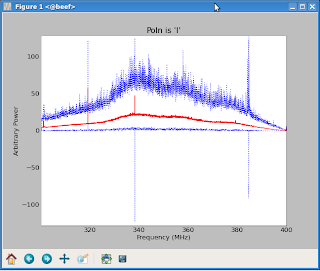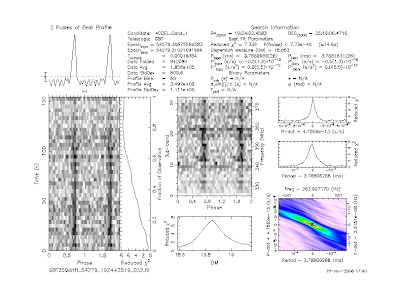### Testing whether a signal is broad-band

Radio pulsars are generally broad-band sources — you can hear them over a very wide range of radio frequencies, from about a hundred megahertz up to, in a few cases, tens of gigahertz. Their emission does change with frequency, decreasing as a power law, but over reasonable bandwidths we expect to see their signal in all frequency channels.Radio-frequency interference ("RFI"), on the other hand, is very frequently narrow-band, appearing in just one, or a few frequency channels. In fact, one way we try to manage RFI is by using really wide bandwidths, so that there's so much power from the pulsar signal that narrow-band RFI is drowned out. Unfortunately, all too often the RFI is so strong that even a narrow-band signal can dominate the total power. And since it's narrow-band, dedispersion doesn't smear it out like it would a broad-band interference spike. So narrow-band RFI is one of the kinds of interference that is particularly hard to sift out from pulsar candidates.

Just recently, on the arxiv, a paper came out that attempts to address the problem of testing whether a candidate is broad-band:
Multimoment Radio Transient Detection
Laura Spitler, Jim Cordes, Shami Chatterjee, Julia Stone
We present a multimoment technique for signal classification and apply it to the detection of fast radio transients in incoherently dedispersed data. Specifically, we define a spectral modulation index in terms of the fractional variation in intensity across a spectrum. A signal whose intensity is distributed evenly across the entire band has a much lower modulation index than a spectrum with the same intensity localized in a single channel. We are interested in broadband pulses and use the modulation index to excise narrowband radio frequency interference (RFI) by applying a modulation index threshold above which candidate events are removed. The technique is tested both with simulations and using data from sources of known radio pulses (RRAT J1928+15 and giant pulses from the Crab pulsar). We find that our technique is effective at eliminating not only narrowband RFI but also spurious signals from bright, real pulses that are dedispersed at incorrect dispersion measures. The method is generalized to coherent dedispersion, image cubes, and astrophysical narrowband signals that are steady in time. We suggest that the modulation index, along with other statistics using higher-order moments, should be incorporated into signal detection pipelines to characterize and classify signals.
This looks very promising, but there's some testing I wish they'd done. More on the subject below the jump.

First of all, "multimoment" makes it sound more impressive than it is: normally, to combine many channels to produce a pulse profile, we simply average them all (after dedispersion). They suggest also computing the "modulation index", which is really just an appropriately normalized standard deviation. The idea is that for the same average power, a narrowband signal will have a much larger standard deviation than a broadband signal. So in addition to keeping only those pulses that have some minimum signal-to-noise when we average all the channels, we would also discard any pulses whose modulation index is too high.The basic idea seems solid to me, but I have some questions. For one thing, we usually have thousands of channels, and the pulse rarely becomes visible until we've averaged together many or all of them. Won't the modulation index be dominated by the noise in each channel rather than the modulation of the real signal? If so, is there some best number of channels to downsample to before computing the modulation index? If there is and the number is small, we might be able to use the modulation index to evaluate our existing pulsar periodicity candidates. So: to the ipython notebook!

Boilerplate:

In :
fr

from IPython.zmq.pylab import backend_inline
cfg = backend_inline.InlineBackendConfig.instance()
cfg.figure_format = 'svg' # 'png' to switch back

import numpy as np
import matplotlib.pyplot as plt
plt.gray();

Utility:

In :
        # rollaxis jiggery-pokery goes here

def downsample(a, factor, axis=0):
    if axis:
        # rollaxis jiggery-pokery goes here
        raise NotImplementedError
    a = np.asarray(a)
    s = a.shape
    ns = (s//factor,factor)+s[1:]
    return np.mean(np.reshape(a,ns),axis=1)

Constructing a fake signal. The parameter "snr" is the signal-to-noise in a single bin of the output profile. The fake signal consists of some bins that have equal power in all channels, some bins that have the same average power but concentrated in one channel, and some bins that have no power. Normal random noise is added to all of them.

In :
snr = 8

channels = 1024
repeats = 64
snr = 8.

signal = np.zeros((channels,3*repeats))
signal[:,:repeats] = 1
signal[channels//2,repeats:2*repeats] = channels
signal += (np.sqrt(channels)/snr) * np.random.randn(*signal.shape)

plt.title("Downsampled channelized view");
plt.imshow(downsample(signal,16),interpolation='nearest');
plt.figure();
plt.title("Mean profile");
plt.plot(np.mean(signal,axis=0));

This computes the "modulation index" as described in the paper. It's really just a normalized standard deviation. They define it using the mean-of-squares minus square-of-mean formula, which is numerically icky, but with only a thousand numbers of modest size it's just not worth coming up with the right normalization for np.std.

In :
        raise NotImplementedError

def modulation_index(a,axis=0):
    if axis:
        raise NotImplementedError
    a1 = np.mean(a**2,axis=axis)
    return (a1-np.mean(a,axis=axis)**2)/a1
In :
pl

plt.plot(modulation_index(signal));
plt.ylabel("Modulation index");

Note that the modulation index is clearly different between the broadband and the narrowband signals. Unfortunately, it's still pretty high for the broadband signal. What happens if we average channels together to improve the signal-to-noise in each channel?

In :
plt.ylabel("Modulation index");

s = signal.copy()
while s.shape>2:
    plt.plot(modulation_index(s), label="%d"%s.shape);
    s = downsample(s,4)
plt.legend(loc="best");
plt.ylabel("Modulation index");

This lowers the broadband signal's modulation index towards zero, but also the narrowband signal's modulation index. Let's see if we can find the point where they are best separated.

In :
    sig += amp*

nc = channels
rr = 1024
sig_idx = []
rfi_idx = []
while nc>1:
    amp = np.sqrt(nc)/snr
    sig = np.ones((nc, rr))
    sig += amp*np.random.randn(*sig.shape)
    sig = modulation_index(sig)
    sig_idx.append((nc,np.mean(sig),np.std(sig)))
    rfi = np.zeros((nc, rr))
    rfi[0,:] = nc
    rfi += amp*np.random.randn(*rfi.shape)
    rfi = modulation_index(rfi)
    rfi_idx.append((nc,np.mean(rfi),np.std(rfi)))
    nc //= 2


In :
plt.gca

plt.errorbar([s for s in sig_idx],
             [s for s in sig_idx],
             yerr=[s for s in sig_idx]);
plt.errorbar([s for s in rfi_idx],
             [s for s in rfi_idx],
             yerr=[s for s in rfi_idx]);
plt.gca().set_xscale('log');
In :
sep = [(

sep = [(nc,(mr-ms)/np.hypot(ss,sr)) for
       ((nc,ms,ss),(ncr,mr,sr)) in zip(sig_idx,rfi_idx)]
plt.semilogx(*zip(*sep));


So there is definitely a best number of channels to distinguish the two, and it's not that large. But the best number probably depends on the signal-to-noise. Let's test this.

In :
        r.append

def distinguish(nc, snr, rr=1024):
    r = []
    nb = np.zeros((nc,rr))
    nb[0,:] = nc
    for s in [np.ones((nc,rr)),nb]:
        s += (np.sqrt(nc)/snr)*np.random.randn(*s.shape)
        m = modulation_index(s)
        r.append((np.mean(m),np.std(m)))
    return (r-r)/np.hypot(r,r)

In :
plt.ylabel("Number of sigma distinction")

nc = [2**(i+1) for i in range(10)]
for snr in [1,2,4,8,16,32]:
    plt.loglog(nc, [distinguish(nci,snr) for nci in nc],
                 label="SNR %g" % snr)
plt.xlim(2,nc[-1])
plt.xlabel("Number of channels")
plt.ylabel("Number of sigma distinction")
plt.legend(loc="best");

So it looks like 8 or 16 channels is best for realistic SNR values, and we only get a reasonably solid distinction for SNR of around 8. For a folded profile this would be per-bin, and so we'd probably want to average together the "on" bins and compare them to the "off" bins.